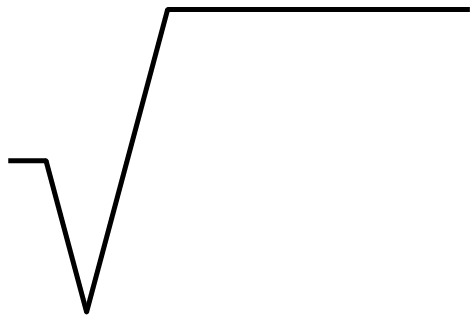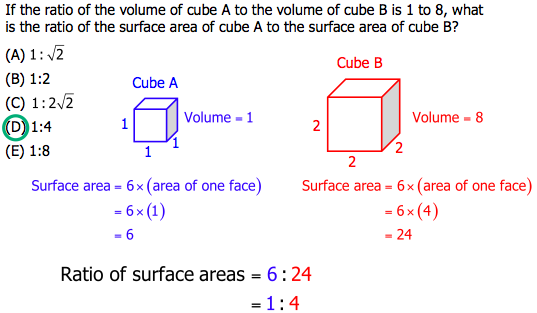# Quant: Roots

## Practice Questions

First, try these practice questions.

Q1. The numbers a, b, and c are all non-zero integers. Is a \gt 0?

Statement #1: a=b^2

Statement #2: a=sqrt{c}

Q2. The numbers a, b, and c are all non-zero integers. Is a \gt 0?

Statement #1: a=b^3

Statement #2: a=\sqrt{c}

Q3. If the ratio of the volume of cube A to the volume of cube B is 1 to 8, what is the ratio of the surface area of cube A to the surface area of cube B?

1:\sqrt{2}

1:2

1:2\sqrt{2}

1:4

1:8

## Square Roots

When you square a number, you are multiplying it by itself, e.g. 6*6 = 36. When you take the square-root of a number, you are undoing the square, going backwards from the result of squaring to the input that was originally squared: \sqrt{36} = 6. Similarly, 8*8 = 64, so \sqrt{64} = 8. As long as all the numbers are positive, everything is straightforward.

It’s easy to find the square root of a perfect square. All other square roots are ugly decimals. For estimation purposes on the very hardest QUANT questions, it might be useful to memorize that \sqrt{2} = 1.4 and \sqrt{3} = 1.7, but without a calculator, no one is going to ask you to calculate the values of any decimal square roots bigger than that. If something like \sqrt{52} shows up, all you have to recognize is between what integers you would find that decimal. For example,

49 \lt 52 \lt 64

therefore

\sqrt{49} \lt \sqrt{52} \lt \sqrt{64}

therefore

7 \lt \sqrt{52} \lt 8

It’s also good to know how to simplify square roots.

## The Symbol: Positive or Negative?What is the name of this symbol? The benighted unwashed masses will call this simply the “square root symbol”, but that’s not the full story. The technical name is the “principal square root symbol.” Here, “principal” (in the sense of “main” or “most important”) means: take the positive root only.

This thickens the plot. The equation x^2 = 16 has two solutions, x = +4 and x = -4, because 4^2 = 16 and (-4)^2 = 16, and the QUANT will impale you for only remembering one of those two. At the same time, \sqrt{16} has only one output: \sqrt{16} = +4 only. When you yourself undo a square by taking a square root, that’s a process that results in two possibilities, but when you see this symbol as such, printed as part of the problem, it means find the positive square root only.

Notice that we can take the square root of zero: 0^2 = 0, so \sqrt{0} = 0, perfectly legal.

Notice, also, we cannot take the square root of a negative — for example, \sqrt{-1} — because that involves leaving the real number line.

## Cubes and Cube Roots

When we raise a number to the third power, 2^3, that is called “cubing” it (because if we had a cube of side = 2, then the “cube” of that number would equal the volume of the cube). Here, 2^3 = 8. A cube root simply undoes this process: \sqrt{8} = 2. As with a square roots, it’s easy to find the cube roots of perfect cubes, and on the QUANT you would never be expected to find an ugly decimal cube root without a calculator.

Cubes and cube roots with negatives get interesting. While 2^3 = 8, it turns out that (-2)^3 = -8. When you multiply two negatives you get a positive, but when you multiply three negatives, you get a negative. More generally, when you multiply any even number of negatives you get a positive, but when you multiply any odd number of negatives, you get a negative. Therefore, when you cube a positive, you get a positive, but if you cube a negative, you get a negative.

This means: while you can’t take the square root of a negative, you certainly can take the cube root of a negative. Undoing the equation (-2)^3 = -8, we get \sqrt{-8} = -2. In general, the cube root of a positive will be positive, and the cube root of a negative will be negative.

It can also be a time-saver to remember the first five cubes:

1^3 = 1

2^3 = 8

3^3 = 27

4^3 = 64

5^3 = 125

You generally will not be expected to recognize cubes of larger numbers. Knowing just these will translate handily into all sorts of related facts: for example, \sqrt{125} = 5 and \sqrt{-125} = -5.

If you remember just this, you will be well-prepare for whatever the QUANT asks you about roots.

## Explanations of the Practice Questions

Q1. All that is given in the prompt is that a, b, and c are non-zero integers.

Statement #1: the result of square anything is always positive, so whether b is negative or positive, a must be positive. This statement, by itself, is sufficient.

Statement #2: since the square root symbol is printed as part of the problem, the output of the \sqrt{c} must be positive. We know for a fact that a must be positive. Again, this statement, by itself, is sufficient.

Both statements are sufficient.

Q2. Again, all that is given in the prompt is that a, b, and c are non-zero integers.

Statement #1: now, if we cube a positive, we get a positive, but if we cube a negative, we get a negative. The numbers a \And b are either both positive or both negative, but since we don’t know the sign of b, we cannot determine the sign of a. This statement, by itself, is insufficient.

Statement #2: if we take the cube root of a positive, we will get a positive, but if we take the cube-root of a negative, we get a negative. The numbers a \And c are either both positive or both negative, but since we don’t know the sign of c, we cannot determine the sign of a. This statement, by itself, is insufficient.

Combined Statements: If we put both statements together, we get that all three numbers, a, b, and c, have to have the same sign: either all three are positive, or all three are negative. We have no further information that would allow us to determine which of those two is the case. Thus, even with combined statements, we still do not have enough information to give a definitive answer to the prompt question. Combined, the statements are still insufficient.